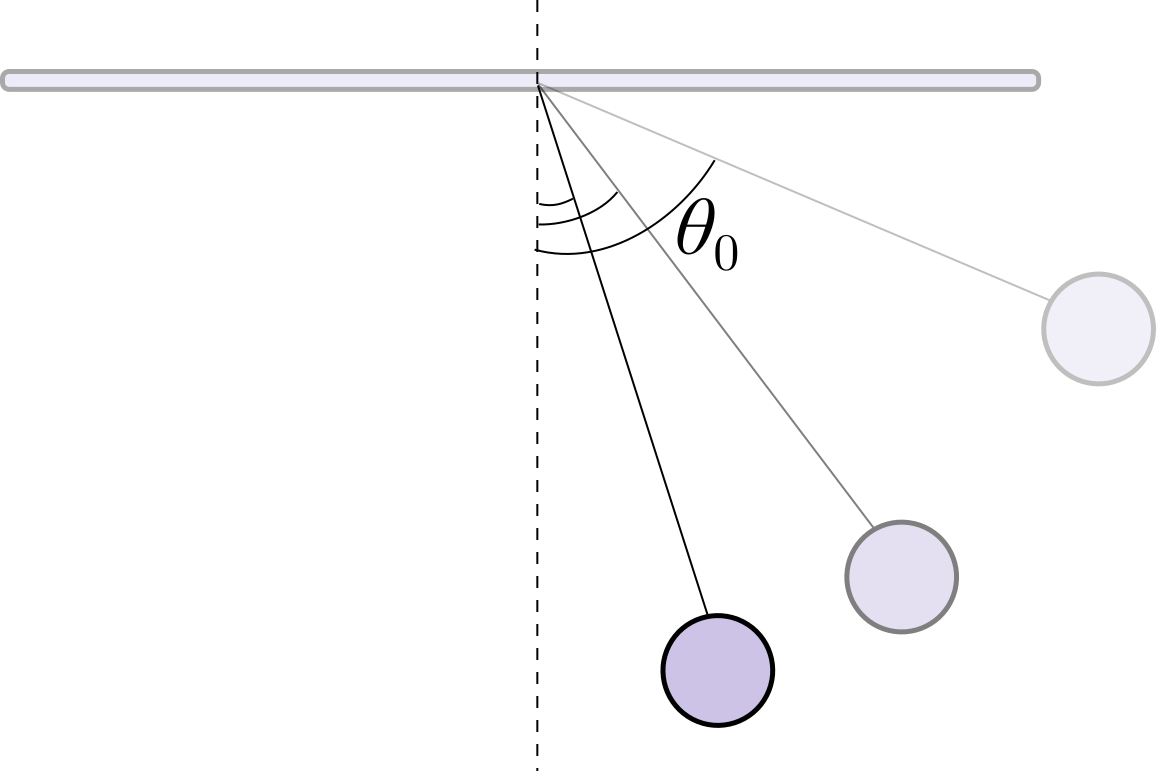# Merrily Swinging Around

When we swing a pendulum with a small angle, we can approximate its motion to be simple harmonic motion. For a pendulum whose length is $l$, the time period of the pendulum is given by $T = 2 \pi \sqrt{\frac{l}{g}}$. Note that the time period is independent of the amplitude of oscillation.

Does this result hold true for larger amplitudes as well? How does the time period depend on the amplitude $\theta_0$ as it goes from $0^\circ$ to $90^\circ?$


Note: Ignore air resistance×